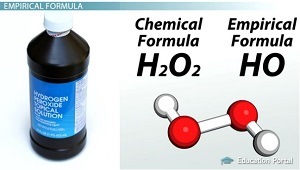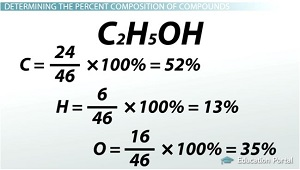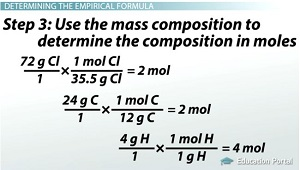# Calculating Percent Composition and Determining Empirical Formulas

An error occurred trying to load this video.

Try refreshing the page, or contact customer support.

Coming up next: Hydrates: Determining the Chemical Formula From Empirical Data

### You're on a roll. Keep up the good work!

Replay
Your next lesson will play in 10 seconds
• 0:07 Empirical Formula
• 2:12 Determining the…
• 3:56 Determining the…
• 5:45 Practice
• 10:16 Lesson Summary
Save Save

Want to watch this again later?

Timeline
Autoplay
Autoplay
Speed Speed

#### Recommended Lessons and Courses for You

Lesson Transcript
Instructor: Amy Meyers

Amy holds a Master of Science. She has taught science at the high school and college levels.

Learn the difference between the empirical formula and chemical formula. Learn how to calculate the percent composition of an element in a compound. Learn how, if given a percent composition, to determine the empirical formula for a compound.

## Empirical Formula

This lesson will cover how to start with an empirical formula and determine the chemical formula. There are several steps to get to the empirical formula. I'm going to break it into two halves. In the first half, you learn how to determine the percent of elements in a compound. In the second part, you learn how to take those percentages and determine the empirical formula.

First though, you need to understand what I mean when I say 'empirical formula.' The empirical formula shows the kind and proportions of atoms in a substance in its simplest form. The chemical formula, on the other hand, is the shorthand way of writing a substance by using chemical symbols and number subscripts with the exact numbers of atoms.

It's like saying the word family. I can tell you that my family consists of a mother, father and some kids. This is like the empirical formula: 1M 1F 2SD (one mother, one father, two sons and daughters).

But, to be more specific, my family consists of a mother, a father, seven sons and three daughters. This is like the chemical formula: 1M 1F 7S 3D (one mother, one father, seven sons, three daughters).

It sounds a bit confusing, but let's look at a few examples. Consider hydrogen peroxide. The chemical formula is H2O2. In one molecule of hydrogen peroxide, there are two hydrogens and two oxygens. The empirical formula is HO. This is how you would write hydrogen peroxide, H2O2, in its simplest form.The chemical formula, as you can tell, gives a more exact representation of the substance. Look at glucose and formaldehyde. Glucose's chemical formula is C6H12O6. Formaldehyde's chemical formula is CH2O. Both of them have the same empirical formula of CH2O.

## Determining the Percent Composition of Compounds

First, let's practice finding the percent composition of compounds. To find the percent composition, you need the formula, from which you find the molar mass (from which you find the mass percentage) of each element.

1. Write the formula.
2. Use the formula to determine molar mass.
3. Use the molar mass to determine the mass percentage of each element.

If you have a compound that has the formula C2H5OH, first determine the mass of each element.

• mass of C = (2 moles C / 1) * (12 g C / 1 mole) = 24 g C
• mass of H = (6 moles H / 1) * (1 g H / 1 mole) = 6 g H

When you are counting the number of hydrogens, don't forget to count the one at the end of the formula!

• mass of O = (1 mole O / 1) * (16 g O / 1 mole) = 16 g O
• Total mass = 24 g + 6 g + 16 g = 46 g

Next, determine the percent of each element in the total compound.

• C = (24 / 46) * 100% = 52%
• H = (6 / 46) * 100% = 13%
• O = (16 / 46) * 100% = 35%## Determining the Empirical Formula

Now, let's practice determining the empirical formula of a compound. To do this, you need the percent composition (which you use to determine the mass composition), then the composition in moles and finally, the smallest whole number mole ratio of atoms.

1. Find the percent composition.
2. Use the percent composition to determine the mass composition.
3. Use the mass composition to determine the composition in moles.
4. Use the composition in moles to find the smallest whole number ratio of atoms.

For this example, our compound has 72% Cl, 24% C and 4% H. First, determine the mass of each of the elements in 100 g of the substance.

• 72 g Cl
• 24 g C
• 4 g H

Next, determine how many moles there are of each element in 100 g of the substance by using the molar mass of each element.

• (72 g Cl / 1) * (1 mole Cl / 35.5 g Cl) = 2 moles
• (24 g C / 1) * (1 mole C / 12 g C) = 2 moles
• (4 g H / 1) * (1 mole H / 1 g H) = 4 moles

Divide each one by the smallest number of moles.

• Cl = 2 / 2 = 1
• C = 2 / 2 = 1
• H = 4 / 2 = 2

So, the empirical formula is CH2Cl.## Practice

Now, let's put it all together. So, pretend you have made something in the lab. You know you reacted 0.35 g of a compound containing carbon, hydrogen and nitrogen with some oxygen, and you made 0.50 g of carbon dioxide and 0.50 g of water. You want to know how many of each atom is in the compound that you started with. In other words, you want to know the empirical formula.

Givens:

• C + H + N + O <==> CO2 + H2O
• 0.35 g = C + H + N
• 0.50 g = CO2
• 0.50 g = H2O

To do this, you need to make a few assumptions. Assume that all of the carbon was used to make the CO2. You have the mass of CO2 (0.50 g). This is how you do it:

• (Mass of carbon / molar mass of CO2) * (mass of CO2 made / 1) = amount of carbon in CO2

To unlock this lesson you must be a Study.com Member.

### Register to view this lesson

Are you a student or a teacher?

#### See for yourself why 30 million people use Study.com

##### Become a Study.com member and start learning now.
Back
What teachers are saying about Study.com

### Earning College Credit

Did you know… We have over 200 college courses that prepare you to earn credit by exam that is accepted by over 1,500 colleges and universities. You can test out of the first two years of college and save thousands off your degree. Anyone can earn credit-by-exam regardless of age or education level.